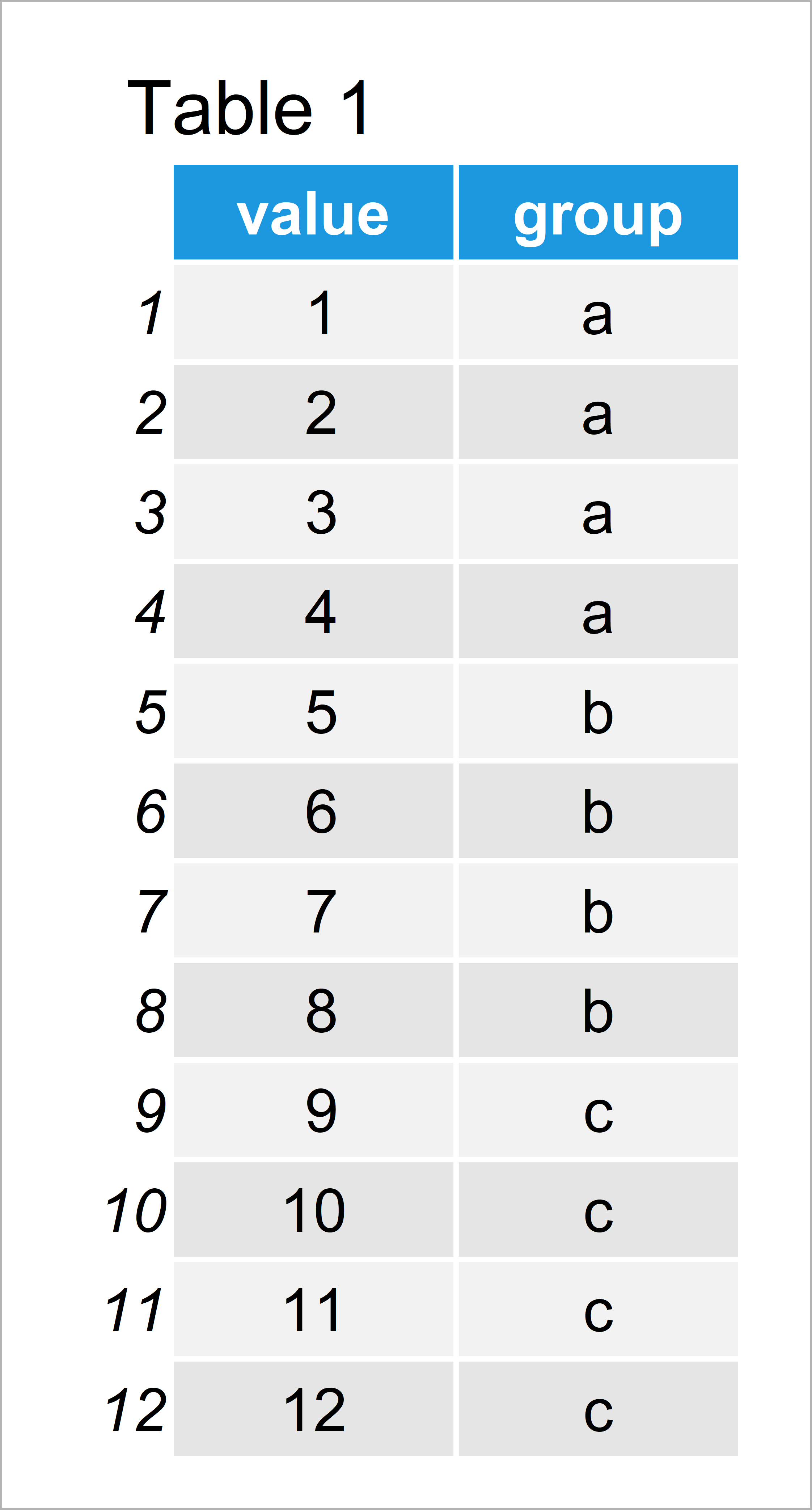# R dplyr group_by & summarize Functions don’t Work Properly (Example)

In this R tutorial you’ll learn how to make the group_by and summarize functions of the dplyr package work properly.

Let’s start right away!

## Creation of Example Data

Consider the example data below:

```data <- data.frame(value = 1:12, # Create example data group = factor(rep(letters[1:3], each = 4))) data # Print example data```Table 1 shows the structure of our example data: It consists of twelve rows and two variables. The column value contains different numeric values and the column group contains a group indicator.

## Example 1: Apply group_by & summarize Functions After Loading plyr Package

This example illustrates why the group_by and summarize functions might not work as expected.

We first need to install and load the dplyr package, in order to use the corresponding functions:

```install.packages("dplyr") # Install dplyr package library("dplyr") # Load dplyr package```

Next, we also have to install and load the plyr package:

```install.packages("plyr") # Install & load plyr package library("plyr")```

Now, we might try to apply the group_by and summarize functions of the dplyr package as shown below:

```data %>% # plyr version group_by(group) %>% summarize(mean = mean(value)) # mean # 1 6.5```

As you can see based on the output of the RStudio console, the previous R code returned only the mean of the entire variable. However, we expected a group version…

The reason for this is that the plyr package also contains a function that is called summarize. Since we have loaded the plyr package after the dplyr package, the R programming language automatically used the plyr version of the function.

So how can we solve this problem? That’s what I’ll explain next!

## Example 2: Apply group_by & summarize Functions with Explicit dplyr Specification

In Example 2, I’ll illustrate how to handle the issue of unexpected outputs when using the group_by and summarize functions of the dplyr package.

As explained in the previous example, the problem is that R automatically uses the plyr version of the summarize function.

We can tell R to use the dplyr version by specifying the name of the package (i.e. dplyr::) in front of the summarize function.

Have a look at the R code below:

```data %>% # dplyr version group_by(group) %>% dplyr::summarize(mean = mean(value)) # # A tibble: 3 x 2 # group mean # <fct> <dbl> # 1 a 2.5 # 2 b 6.5 # 3 c 10.5```

As you can see, now the group_by and summarize functions work fine.

## Video & Further Resources

Have a look at the following video of my YouTube channel. In the video, I’m explaining the R programming codes of the present tutorial in the R programming language.

Summary: In this tutorial you have learned how to make the dplyr group_by and summarize functions work in the R programming language. Note that similar errors may occur when using other packages where functions have the same name such as ggplot2.

Subscribe to the Statistics Globe Newsletter

•Andrew
February 10, 2021 11:10 am

Great content. Just wanted to add that the “conflicted” package is another notable solution if you don’t want to always name the package before the function

• Hey Andrew,

Thanks for the kind words and for sharing this additional solution! Indeed, it’s also possible to detach the package you don’t want to use 🙂

Regards,

Joachim

•Jose
January 27, 2022 10:01 pm

I have run into a similar problem. Even after specifying dplyr::group_by and dplyr::summarize the output that is returned does not provide the grouping instead it provides the original dataset.